# Test: Electronic Devices & Digital Communications - 2

## 20 Questions MCQ Test GATE ECE (Electronics) 2023 Mock Test Series | Test: Electronic Devices & Digital Communications - 2

Description
Attempt Test: Electronic Devices & Digital Communications - 2 | 20 questions in 60 minutes | Mock test for Electronics and Communication Engineering (ECE) preparation | Free important questions MCQ to study GATE ECE (Electronics) 2023 Mock Test Series for Electronics and Communication Engineering (ECE) Exam | Download free PDF with solutions
QUESTION: 1

### A sample of germanium is doped to the extent of 1014 donor atoms/cm3 and 7 x 1013 acceptor atoms/cm3. The resistivity of pure germanium is 60 Ω cm. If the applied electric field is 2V/cm, then the total conduction current density is given by

Solution: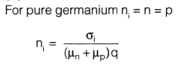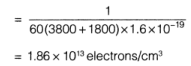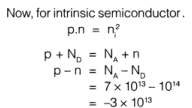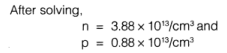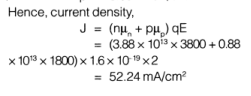QUESTION: 2

### An abrupt p-n junction diode is formed with two sides of resistivities 2 Ω cm in the p-side and 1 Ω cm on the n-side. The height of potential energy barrier for Ge (germanium) is

Solution: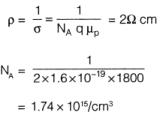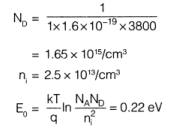QUESTION: 3

### An ideal p-channel MOSFET has the parameters. W = 15 μm, μp = 300 cm2A/ sec, L = 15 [μm, tox = 350 A° and VT = -0.80 V. If transistor is operating in non saturation region at VSD = 0.5 V, then the value of gm is ____________ μS

Solution: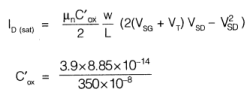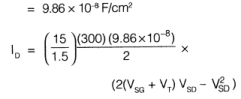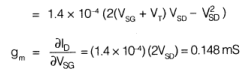QUESTION: 4

A Varactor diode has depletion capacitance given by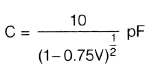where V is the bias voltage on the diode in volts. The diode is placed in parallel with a 0.75 (μH inductor which forms part of the frequency modulator. The required bias voltage so that the inductor diode combination resonates at 100 MHz is given by

Solution: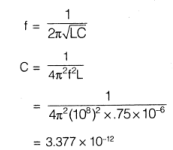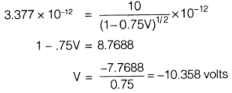QUESTION: 5

Figure shown is a battery charging circuit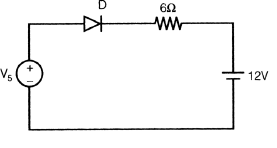If Vs = 120 sinωt, the conduction angle of the diode D is given by (i.e. in positive half cycle for how much total angle it conducts)

Solution: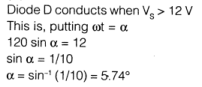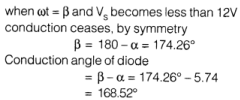QUESTION: 6

If the magnitude of the zener voltage is 4.9 V, what will be the current in the circuit? (The reverse saturation current is 5 μA).

Solution:
QUESTION: 7

In the BJT current source shown in figure, the diode voltage and the transistor base to emitter voltage are equal. If base current is neglected then collector current is __________ mA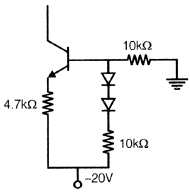Solution: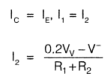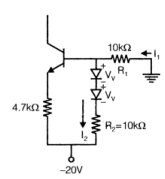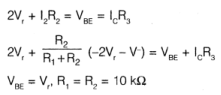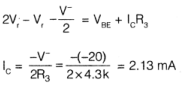QUESTION: 8

Two p-n germanium diodes are connected in series opposing. A 5V battery is impressed upon this series arrangement.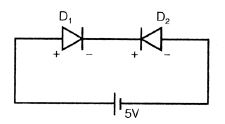The voltage across reverse bias p-n junction (Assume that the magnitude of the zener voltage is greater than 5V.

Solution: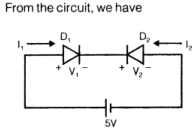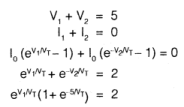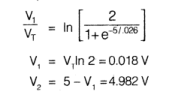QUESTION: 9

The parameters of an FET are gm = 3 mA/V, rd = 30 k, RL = 3k as an source follower load. The output impedance is given by

If R2 is replaced by a capacitor, then circuit behaves as

Solution: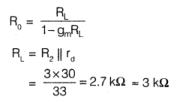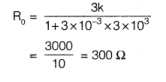QUESTION: 10

The N-channel FET having lDSS = 1 mA Vp = -1 V. The value of R1 when quiescent drain to ground voltage is 10 V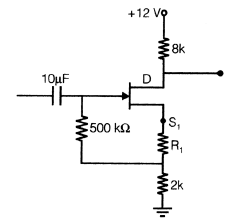Solution: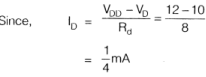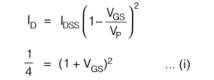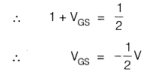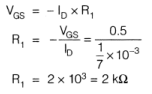QUESTION: 11

Diode current in circuit below for R = 20 kΩ is? When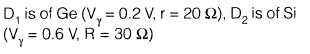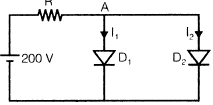Solution: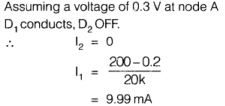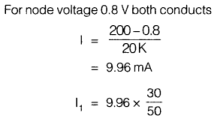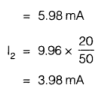QUESTION: 12

The ramp signal m(t) = at is applied to a delta modulator with sampling period “Ts” and step size “δ” then slope over load distortion would occur if,

Solution: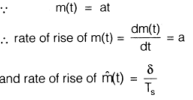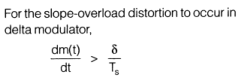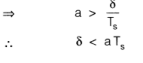QUESTION: 13

Consider the transistor circuit given below having an ideal diode: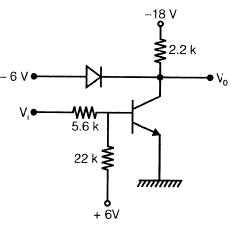Q.

The minimum value of hfe required neglecting saturation operation and assuming an ideal diode is

Solution: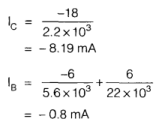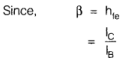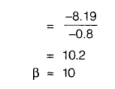QUESTION: 14

Consider the transistor circuit given below having an ideal diode:Q.

The maximum temperature at which the inverter would operate properly if the reverse saturation current at 25°C is 5 |xA is

Solution: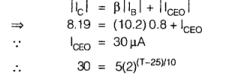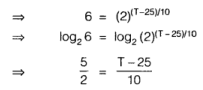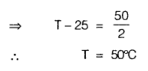QUESTION: 15

In a PCM system , if the quantization levels are increased from 2 to 16, the relative BW requirement will

Solution: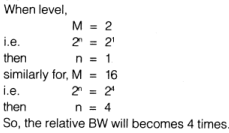QUESTION: 16

A random variable has following probability function.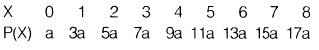Q.

Value of a is

Solution:
QUESTION: 17

A random variable has following probability function.Q.

Value of P (X < 4)

Solution:
QUESTION: 18

For a low pass filter circuit shown below if auto correlation function of input process x(t) is given by,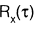= 5 δ(t) then,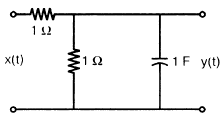Q.

PSD of the output random process is

Solution: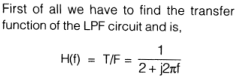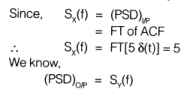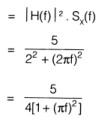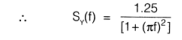QUESTION: 19

For a low pass filter circuit shown below if auto correlation function of input process x(t) is given by,= 5 δ(t) then,Q.

Average power of output random process is

Solution: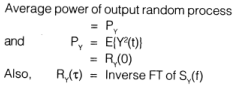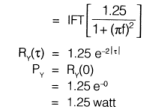QUESTION: 20

E(x) for joint probability function of 2 discrete random variables x and y given as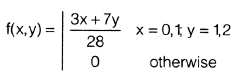Solution: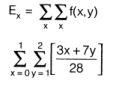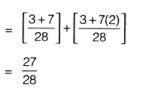Use Code STAYHOME200 and get INR 200 additional OFF Use Coupon Code